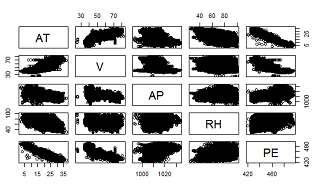# Regression Analysis using R

What is a Prediction Problem?
A business problem which involves predicting future events by extracting patterns in the historical data. Prediction problems are solved using Statistical techniques, mathematical models or machine learning techniques.
For example: Forecasting stock price for the next week, predicting which football team wins the world cup, etc.

What is Regression analysis, where is it applicable?
While dealing with any prediction problem, the easiest, most widely used yet powerful technique is the Linear Regression. Regression analysis is used for modeling the relationship between a response variable and one or more input variables.
In simpler terms,Regression Analysis helps us to find answers to:
• Prediction of Future observations
• find association, relationship between variables.
• Identify which variables contribute more towards predicting the future outcomes.Types of regression problems:

#### Simple Linear Regression:

If model deals with one input, called as independent or predictor variable and one output variable, called as dependent or response variable then it is called Simple Linear Regression. In this type of Linear regression, it assumes that there exists a linear relation between predictor and response variable of the form.
In the above equation, β0,β1 are the unknown constants that represent intercept and slop of a straight line which we learned in our high schools. These known constants are known as the model coefficients or parameters. From the above equation, X is the known input variable and if we can estimate β0,β1 by some method then Y can be predicted. In order to predict future outcomes, by using the training data we need to estimate the unknown model parameters (ˆ β0,ˆ β1) using the equation.
ˆy = ˆ β0 + ˆ β1x + ˆe, where ˆ y,ˆ β0,ˆ β1 are the estimates.
Multiple Linear Regression:
If the problem contains more than one input variables and one response variable, then it is called Multiple Linear regression.

How do we apply Regression analysis using R?
Let us apply regression analysis on power plant dataset available from here. The dataset contains 9568 data points collected from a Combined Cycle Power Plant over 6 years (2006-2011), when the power plant was set to work with full load. Features consist of hourly average ambient variables Temperature (T), Ambient Pressure (AP), Relative Humidity (RH) and Exhaust Vacuum (V) to predict the net hourly electrical energy output (EP) of the plant.
• Read the data into R environment:
`require(xlsx)`
`sample1 = read.xlsx("C:\\Suresh\\blogs\\datasets\\CCPP\\Folds5x2_pp.xlsx",sheetIndex=1)`
•  Understand and observing the data: View(sample1)
Check for missing values, range of variables, density plots for each of the varaible:
` `
`sum(is.na(sample1\$AT))`
` 0`
`range(sample1\$AT) #1.81,37.11`
`mean(sample1\$AT) #m: 19.65`
`plot(density(sample1\$AT))`
`Plot(sample1)`
``
`Density plot for Temperature`
``
` `

`Scatter plots shows us that temperature (AT) and vaccum(V) are inversely related to power while pressure(AP) and RH are not related.`
•  Check for correlation among the variables. This step is very important to understand the relation of dependant variable with the independent variables and correlations among the variables. In general, there shouldn’t be any correlation among the independent variables.
cor(sample1)
`'AT          V          AP          RH         PE`
`AT  1.0000000  0.8441067 -0.50754934 -0.54253465 -0.9481285`
`V   0.8441067  1.0000000 -0.41350216 -0.31218728 -0.8697803`
`AP -0.5075493 -0.4135022  1.00000000  0.09957432  0.5184290`
`RH -0.5425347 -0.3121873  0.09957432  1.00000000  0.3897941'`
`'inferences--> AT has -ve relation with PE`
`V is highly related to PE`
`other two are relatively related'`
```
Divide the data into training and test set and train the model with linear regression using lm()  method available in R and thendo predictions on new test data using predict() method.

```
rand=sample1[sample(nrow(sample1)),]
tr = rand[1:6697,]
ts = rand[6698:9568,]
model2 = lm(PE~AT+V+AP+RH,data=tr)
summary(model2)

Call:
lm(formula = PE ~ AT + V + AP + RH, data = tr)

Residuals:
Min      1Q  Median      3Q     Max
-43.533  -3.170  -0.068   3.229  17.451

Coefficients:
Estimate Std. Error  t value Pr(>|t|)
(Intercept) 457.729155  11.794172   38.810  < 2e-16 ***
AT           -1.987307   0.018208 -109.147  < 2e-16 ***
V            -0.231996   0.008692  -26.689  < 2e-16 ***
AP            0.059235   0.011442    5.177 2.32e-07 ***
RH           -0.159916   0.005015  -31.886  < 2e-16 ***
---
Signif. codes:  0 ‘***’ 0.001 ‘**’ 0.01 ‘*’ 0.05 ‘.’ 0.1 ‘ ’ 1
Residual standard error: 4.585 on 6692 degrees of freedom
Multiple R-squared:  0.9281,      Adjusted R-squared:  0.9281
F-statistic: 2.161e+04 on 4 and 6692 DF,  p-value: < 2.2e-16

New predictions are made using predict method.

The above image shows the results of actual vs predicted which are quite accurate. In the summary results of  the model, below are the key takeways:
• Model is accurate as R2 is near to 1 (0.912).
• Model states all the variables are significant, the ***  indicate the significance.
•  P-statistics is less than 0.05, F-statistics is significantly high.
• Residuals vs fitted plots and Q-Q normal plots are also good with mean variance of the errors around 0.

In the next blog we learn about model validations and extensions in linear regression.

1.However, its additional potential does not end here. AI can likewise support representative commitment by improving the organization culture. machine learning course

2.LifeVoxel AI - Best Medical Imaging Platform also Provide critical patient-centric delivery of care using intuitive, diagnostic and instantly accessible visualizations over the Internet.

RIS PACS
RIS PACS Software

3.data science training in noida

4.This post is very simple to read and appreciate without leaving any details out. Great work!
5.6.